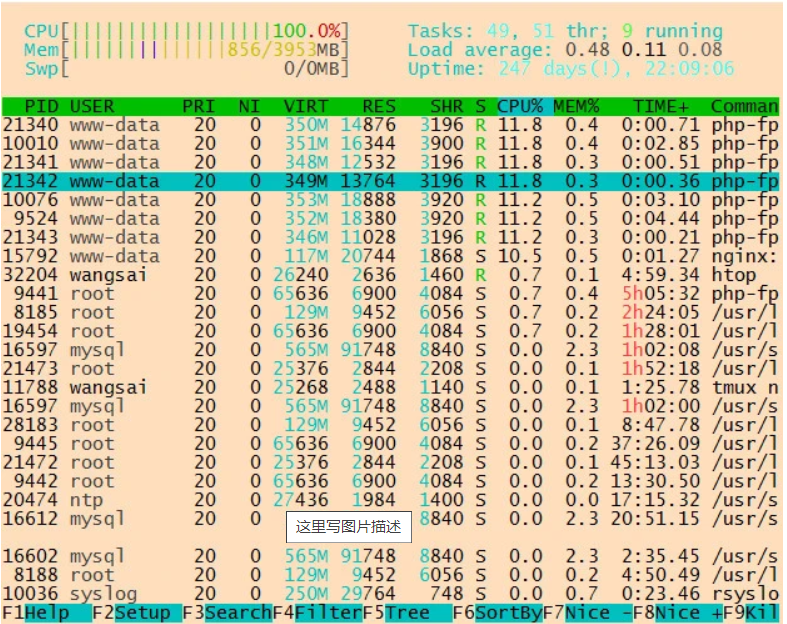# php优化

php源码安装.configure时指定mysqlnd驱动，老版本php源码安装时需要指定mysql安装的相关几个地址

3     public function rstoarray(&$result,$field = '')
4     {
5         $arrRs =$result->fetch_all(MYSQLI_ASSOC); //该函数只能用于php的mysqlnd驱动
6         $result->free(); 7 8 if ($field) {
9             $arrResult = []; 10 foreach ($arrRs as $v) { 11$arrResult[$v[$field]] = $v; 12 } 13 return$arrResult;
14         }
15
16         return $arrRs; 17 } 图中有一个水平线，内存没有增加，但是耗费了很多时间 那里的代码是一段排序操作，用到了php的usort(); 自定义排序， 需要自己写一个排序用的回调函数， 这段效率特别低 优化： [ 'a' => ['sort' => 123], 'b' => ['sort' => 234] ..... ] 先遍历要排序的二维数组(如上边的那个)， 生成一个一维数组， 键为二维数组的键，值为排序用的那个值: [ 'a' => 123, 'b' => 234 ] 然后用php的排序函数： asort() 或者 arsort();对这个一维数组排序， 然后根据对应关系生成一个新的完整的数组， 这样会快很多  1 //按照二维数组中的某个键进行排序 2 public function mysort(&$arr, $key,$desc = '')
3     {
4         $tmp = array(); 5 foreach ($arr as $k=>$v) {
6             $tmp[$k] = $v[$key];
7         }
8
9         if ($desc) { 10 arsort($tmp);
11         } else {
12             asort($tmp); 13 } 14 15$result = array();
16         foreach ($tmp as$k => $v) { 17$result[$k] =$arr[$k]; 18 } 19 20 return$result;
21     }

SummerPHP 框架18 033703 048 条高效率的 PHP 优化写法
48 条高效率的 PHP 优化写法
44 0php + nginx 网站并发压力测试及优化

209 0171 01125 0php使用tcp长连接的一种优化思路

755 0Nginx PHP配置与优化(学习笔记二十二)

1088 0947 0
mydcool
php相关专家

PHP完全自学手册文档教程946088

PHP 2017.北京 全球开发者大会——高可用的PHP